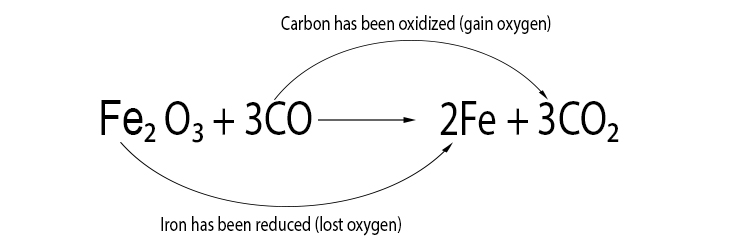# Redox Reactions – Reduction and oxidation

Oxidation: An atom loses an electron in a chemical reaction (sometimes, oxygen is gained)
Reduction: An atom gains an electron in a chemical reaction (sometimes, oxygen is lost. Sometimes hydrogen is acquired)

Note: If a neutral atom loses an electron it becomes positively (+) charged. If a neutral atom gains an electron, it becomes negatively (–) charged.

For example, sodium can lose an electron in a reaction to become Na⁺, a positive sodium ion. We can say the sodium has been oxidised.

Chlorine (Cl) can gain an electron in a reaction to become negatively charged Cl⁻, a negatively charged ion. We can say the chlorine atom has been reduced to form a chloride ion.

If the +ve element and –ve element immediately attract and form another compound, this is called a redox reaction.

A redox reaction is a reaction that involves the transfer of electrons between elements and then an immediate bond.

The word 'REDOX' is made from words that describe the two reactions: reduction and oxidation.

In an oxidation reaction, a metal loses electrons and forms an ionic bond with oxygen that has stolen the electrons.

(Note: this is why oxidation is sometimes referred to as the gain of oxygen by original scientists but now we understand electrons, we know it occurs with other elements).

In a reduction reaction, oxygen can leave an element like carbon and gain an electron from a metal and then join with the metal to form an ionic bond.

(Note: this is why reduction is sometimes referred to as the loss of oxygen because an element has lost oxygen).

The best way to remember this is to recall the following mnemonic:Oxidation is the loss of electrons and reduction is the gain of electrons.

To remember this think OIL RIG.

This stands for:

Oxidation Is the Loss of electrons

Reduction Is the Gain of electrons

Also remember this mnemonic:

An element that gains oxygen is oxidation. An element that loses oxygen has a reduced amount of oxygen is reduction (and therefore an element that loses oxygen is reduction).

### Example 1

Iron oxide + carbon monoxide → iron + carbon dioxide### Example 2

Magnesium + oxygen → Magnesium oxide

2Mg + O2 → 2MgO

If you are given this equation and asked which substance has reduced and which has oxidised, you don’t need worry about the electrons. You can just say:

Magnesium → Magnesium oxide

2Mg → 2MgO

Magnesium has gained oxygen and oxidation has taken place. The magnesium has been oxidised.

### Example 3

Copper oxide + hydrogen → copper + water

CuO + H2 → Cu + H2O

If you are given this equation and asked which substance has reduced and which has oxidised, you don’t need to look at the electron arrangement, you can just say:

Copper oxide → Copper

CuO → Cu

Reduction has taken place as copper oxide has lost oxygen.

Copper oxide has been reduced to copper.

### Example 4

2PbO + C  → CO2 + 2Pb

If you are given this equation and asked which substance has reduced and which has oxidised, because it includes oxygen, you can say:

2PbO → 2Pb

Lead (Pb) has lost oxygen and been reduced.

Carbon → Carbon dioxide

C → CO2

On the other hand, carbon has gained oxygen; it has been oxidised.

Lead oxide has been reduced, carbon has been oxidised.